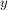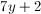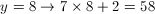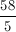# PSAT Math : How to divide integers

## Example Questions

### Example Question #1738 : Sat Mathematics

Kacey works 5 hours per day on Monday, Tuesday, and Wednesday, as well as 9 hours on Thursday and Friday. She does not work on Saturday or Sunday. Her total earnings per week is $363. How much does she earn per hour in dollars? Possible Answers: 9 12 8 11 Correct answer: 11 Explanation: Kacey works 5 hours a day for 3 days and 9 hours a day for 2 days. (5)(3) = 15 and (2)(9) = 18. 15 + 18 = 33 hours worked a week.$363/33 hours = \$11/hr.

### Example Question #1 : Operations

When k is divided by 2, the remainder is 1. If k is divided by 3, the remainder is 0. And if k is divded by 5, the remainder is 3. Which of the following is a possible value for k?

23
57
63
30
27
Explanation:

Since the remainder when k is divided by 2 is 1, this means that k is not an even number. Therefore, we can immediately eliminate 30 from our possible answer choices.

Because the remainder when k is divded by 3 is 0, we know that k must be a multiple of 3. This means we can eliminate 23 from our answer choices.

The only choices left are 63, 27, and 57. When 27 and 57 are divded by five, the remainder is two. Thus, only 63 works, because when 63 is divded by five, the remainder is three.

### Example Question #1 : How To Divide Integers

The result of a number divided by 2 is the same as the result of that number divided by 10. What is that number?

10

1

2

0

0

Explanation:

0 is the only choice that yields the same answer (0) when divided by 2 and 10. You can check this easily by plugging a few of the answer choices into a fraction:

1/2 ≠ 1/10

2/2 ≠ 2/10 etc.

### Example Question #2 : How To Divide Integers

If x and y are positive integers and 2y = 16x, what is the value of y/x?

4

2

1/2

1/4

4

Explanation:

Making the equation even yields y = 4 and x = 1, since 24 = 16. This makes y/x = 4.

### Example Question #861 : Arithmetic

Ifis an integer, and 3 is the remainder whenis divided by 5, then which of the following is a possible value of?

8

2

1

5

9

WhenThe remainder ofis 3 (the answer is 11, remainder 3), sois a possible answer choice. If you try the other answers they won't work.